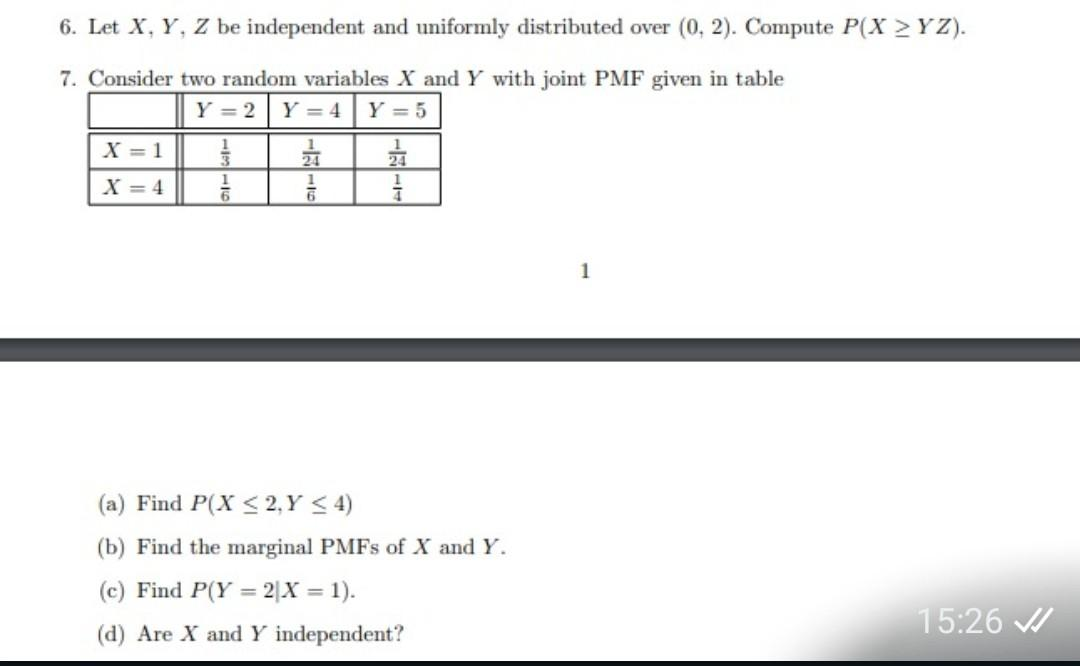# Question Solved1 Answer6. Let X, Y, Z be independent and uniformly distributed over (0, 2). Compute P(x > YZ). 7. Consider two random variables X and Y with joint PMF given in table Y = 2 Y = 4 Y = 5 X = 1 } X = 4 be i 6 1 (a) Find P(X < 2, Y < 4) (b) Find the marginal PMFs of X and Y. (c) Find P(Y = 2 X = 1). (d) Are X and Y independent? 15:26 VFCDMLD The Asker · Probability and StatisticsTranscribed Image Text: 6. Let X, Y, Z be independent and uniformly distributed over (0, 2). Compute P(x > YZ). 7. Consider two random variables X and Y with joint PMF given in table Y = 2 Y = 4 Y = 5 X = 1 } X = 4 be i 6 1 (a) Find P(X < 2, Y < 4) (b) Find the marginal PMFs of X and Y. (c) Find P(Y = 2 X = 1). (d) Are X and Y independent? 15:26 V
More
Transcribed Image Text: 6. Let X, Y, Z be independent and uniformly distributed over (0, 2). Compute P(x > YZ). 7. Consider two random variables X and Y with joint PMF given in table Y = 2 Y = 4 Y = 5 X = 1 } X = 4 be i 6 1 (a) Find P(X < 2, Y < 4) (b) Find the marginal PMFs of X and Y. (c) Find P(Y = 2 X = 1). (d) Are X and Y independent? 15:26 V